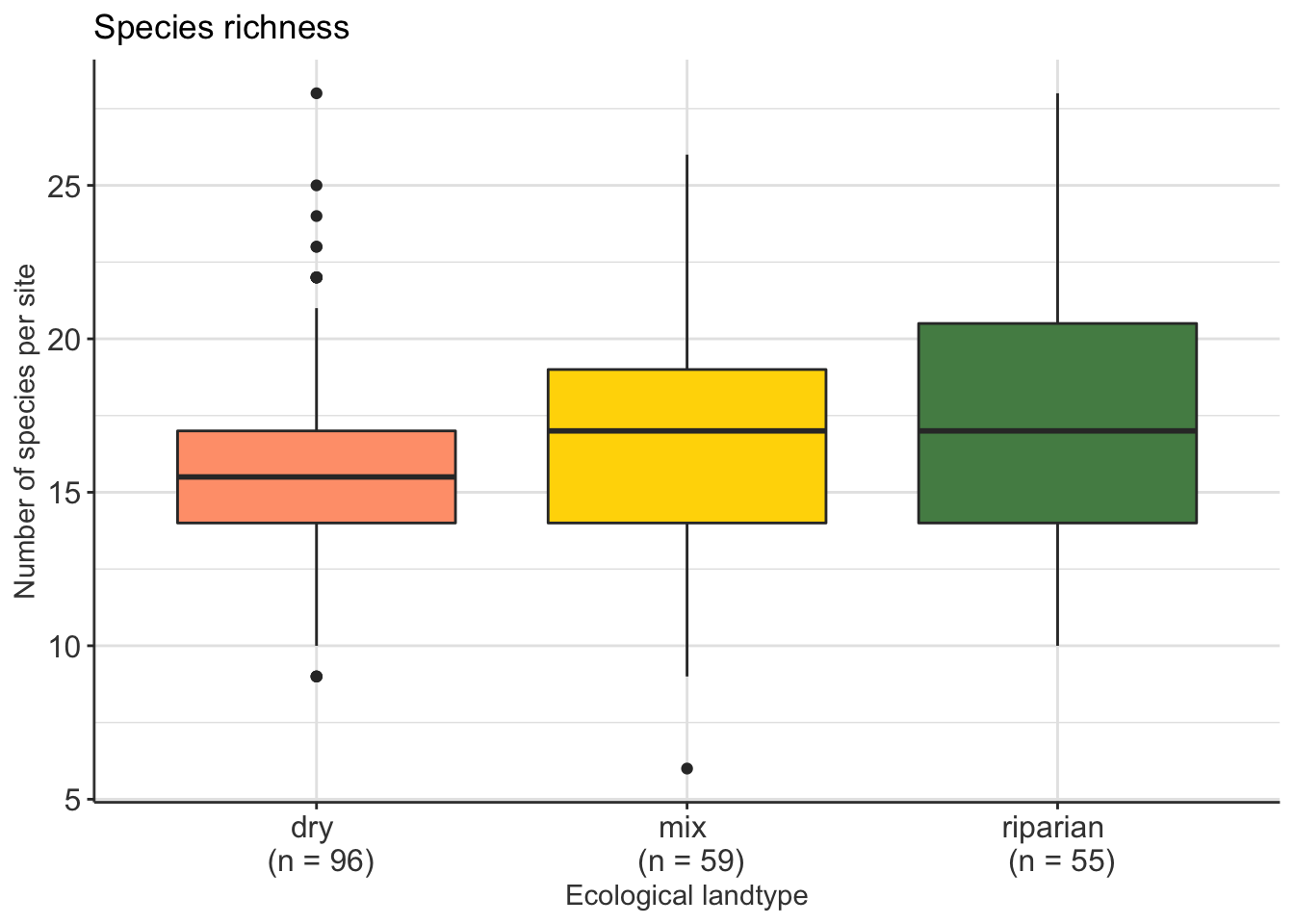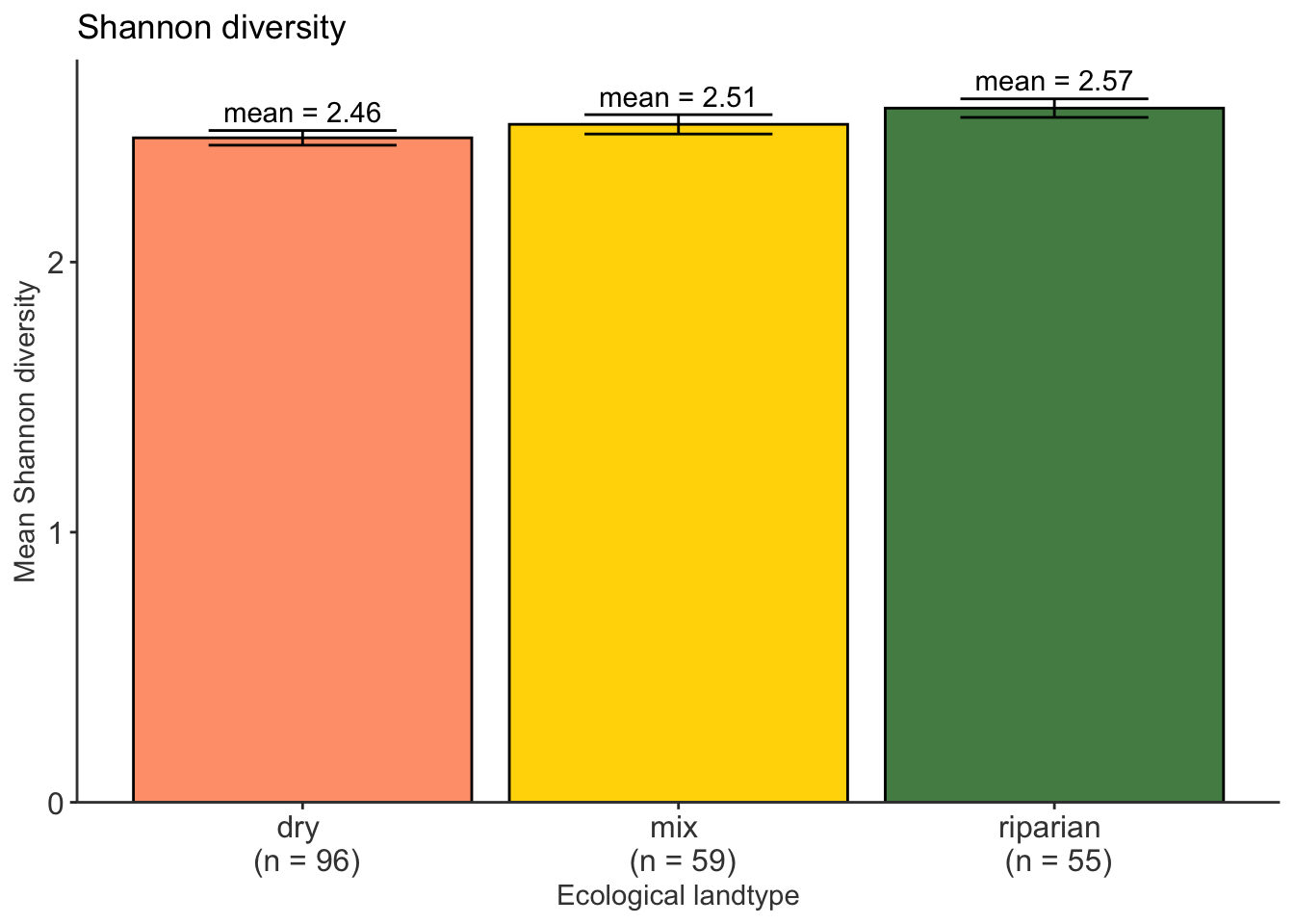### Introduction and main takeaways

In this workshop, we worked through real data on bird communities in three different habitat types. The data are published in a really cool paper: Adams, Bryce T. and Stephen N. Matthews. “Diverse temperate forest bird assemblages demonstrate closer correspondence to plant species composition than vegetation structure.” Ecography. https://doi.org/10.1111/ecog.04487

With the functions from this workshop, you’ll be able to answer the following questions about the data (and your own!):

• How speciose are my communities? specnumber()
• How diverse are my communities? diversity()
• How different are my communities in species composition? adonis(), rda(), metaMDS()
• How do species drive the separation of my communities? envfit()
• How is community structure related to specific environmental variables? cca()

# libraries
library(tidyverse)
library(vegan)
library(ggvegan)

# data
# bird communities
column_to_rownames("site")

# environmental variables

# set up a "metadata" frame - will be useful for plotting later!
site_type <- env %>%
select(site, landtype)

#### What’s all this data?

Bird communities were sampled using point counts at 120 points distributed amongst 3 ecological landtypes: 1. dry (ridgetops), 2. riparian (bottomlands), and 3. mixed (hillslopes). The landtypes can be distinguished using their most common tree species, though the site is speciose (~75 tree species).

The birds data frame is in the format that data generally have to be in to start doing any analysis, which is to say that samples (or sites) are in rows, and species are columns. The only entries in the data frame are counts.
If you’d like to explore what species are in this set, you can translate alpha codes to common names using this chart.

head(birds, 3)
##        ACFL AMCR AMGO AMRE AMRO BAOR BAWW BGGN BHCO BLBW BLJA BRTH BTNW BWWA
## site_1    0    0    1    0    0    0    1    0    0    0    1    0    0    0
## site_2    9    0    0    0    3    0    2    2    1    0    1    0    0    0
## site_3    4    0    0    0    3    0    0    4    4    0    0    0    0    0
##        CACH CARW CEDW CERW CHSP COYE EAPH EATO EAWP ETTI FISP GCFL GRCA HOWA
## site_1    0    0    0    0    0    0    0    1    2    0    0    0    0    7
## site_2    0    0    0    0    0    0    1    0    0    0    0    0    0    4
## site_3    0    0    0    3    0    0    0    1    0    2    0    0    0    2
##        INBU KEWA LOWA NOCA NOPA OVEN PIWA PRAW RBGR REVI RWBL SCTA SUTA WBNU
## site_1    0    0    0    0    0    6    0    0    0   12    0    0    0    1
## site_2    0    0    0    1    0    6    0    0    0    6    0    4    0    0
## site_3    0    0    0    0    0    8    0    0    0    9    0    3    0    3
##        WEVI WEWA WOTH YBCH YTVI YTWA
## site_1    0    0    0    0    1    0
## site_2    0    2    7    0    1    0
## site_3    0    0    6    0    4    0

The env data frame contains all the “environmental” variables that were measured, which in this case was tree community composition and canopy structure. An explanation of the columns is below:

Column Meaning
site point count (1-120)
landtype ecological landtype (dry, riparian, mixed)
stems_ha total stem density (# of stems per hectare) of both under and overstory stems
big_stem_bas large stem basal area (m2/hectare) of trees [stems/trees >/=8 cm diameter at breast height (DBH)]
canopy_cover percent canopy cover
canopy_height mean canopy height (m)

### How speciose are my communities?

specnumber() will tell you the number of species within each sample. You can then run an analysis of variance to ask if mean species richness is significantly different across sites.

sppr <- specnumber(birds)

# analysis of variance takes the same form as the usual models you'd see in R
# response ~ dependent, data = environmental grouping
sppr_aov <- aov(sppr ~ landtype, data = site_type)
summary(sppr_aov)
##              Df Sum Sq Mean Sq F value Pr(>F)
## landtype      2     86    43.0   2.774 0.0648 .
## Residuals   207   3209    15.5
## ---
## Signif. codes:  0 '***' 0.001 '**' 0.01 '*' 0.05 '.' 0.1 ' ' 1

Looks like there’s no significant difference in species richness between landtypes. Let’s plot!

sppr_df <- sppr %>%
enframe() %>%
full_join(site_type, by = c("name" = "site"))

pal <- c("lightsalmon1", "gold1", "palegreen4")

plot_sppr <- ggplot(sppr_df, aes(x = landtype, y = value, fill = landtype)) +
geom_boxplot() +
scale_fill_manual(values = pal) +
scale_x_discrete(labels = c("dry \n (n = 96)", "mix \n (n = 59)", "riparian \n (n = 55)")) +
theme(legend.position = "none",
plot.background = element_rect("white"),
panel.background = element_rect("white"),
panel.grid = element_line("grey90"),
axis.line = element_line("gray25"),
axis.text = element_text(size = 12, color = "gray25"),
axis.title = element_text(color = "gray25"),
legend.text = element_text(size = 12)) +
labs(x = "Ecological landtype",
y = "Number of species per site",
title = "Species richness")
plot_sppr### How diverse are my communities?

There are many diversity metrics out there, but I’ve only included Shannon diversity here, takes into account species abundance and evenness: $H = -\sum_{i=1}^{R}p_iln(p_i)$ where $$R$$ is total richness, $$p_i$$ is the proportion of $$R$$ of the $$i$$th species. The diversity() function in vegan will calculate Shannon, Simpson, and Fisher’s alpha - just select which one you want in the arguments. Here, I’ll calculate Shannon diversity for each site, then plot mean Shannon diversity per landtype.

shannondiv <- diversity(birds)
head(shannondiv)
##   site_1   site_2   site_3   site_4   site_5   site_6
## 1.812353 2.443171 2.502177 2.449965 2.505759 2.508055

You can do the same analysis of variance on Shannon diversity.

sppdiv_aov <- aov(shannondiv ~ landtype, data = site_type)
summary(sppdiv_aov)
##              Df Sum Sq Mean Sq F value Pr(>F)
## landtype      2  0.399 0.19958   2.817 0.0621 .
## Residuals   207 14.668 0.07086
## ---
## Signif. codes:  0 '***' 0.001 '**' 0.01 '*' 0.05 '.' 0.1 ' ' 1

No significant difference in diversity either. Another plot, now in column form.

shandiv_df <- shannondiv %>%
# put all those calculations into a data frame
enframe() %>%
# rename columns for ease of joining
rename(site = name,
shan_div = value)

div_plot_df <- shandiv_df %>%
# join with site_type
full_join(site_type, ., by = "site") %>%
# group by landtype
group_by(landtype) %>%
# calculate mean and standard error of diversity
summarize(mean = round(mean(shan_div), 2),
err = sd(shan_div)/sqrt(length(shan_div))) %>%
dplyr::mutate(label = "mean") %>%
unite("mean_label", label, mean, sep = " = ", remove = FALSE)

clean_background <- theme(plot.background = element_rect("white"),
panel.background = element_rect("white"),
panel.grid = element_line("white"),
axis.line = element_line("gray25"),
axis.text = element_text(size = 12, color = "gray25"),
axis.title = element_text(color = "gray25"),
legend.text = element_text(size = 12),
legend.key = element_rect("white"))

plot_shandiv <- ggplot(div_plot_df, aes(x = landtype, y = mean, fill = landtype)) +
geom_col(color = "black") +
scale_fill_manual(values = pal) +
geom_errorbar(aes(ymin = mean - err, ymax = mean + err), width = 0.5) +
geom_text(aes(x = landtype, y = mean + err + 0.07, label = mean_label)) +
scale_x_discrete(labels = c("dry \n (n = 96)", "mix \n (n = 59)", "riparian \n (n = 55)")) +
scale_y_continuous(limits = c(0, 2.75), expand = c(0,0)) +
clean_background +
theme(legend.position = "none") +
labs(x = "Ecological landtype",
y = "Mean Shannon diversity",
title = "Shannon diversity")
plot_shandiv### How different are my communities in species composition?

#### Permutational Multivariate Analysis of Variance (perMANOVA)

Assessing differences in community composition is done with permutational Multivariate Analysis of Variance, or perMANOVA. These tests are done on distances, meaning that they assess the differences between communities based on dissimilarity. With perMANOVA, the null hypothesis is that the centroids of your groups (in ordination space as defined by the dissimilarity measure you’ve chosen) are equivalent for all groups. In other words, you are asking if, following some measure of (dis)similarity, the community composition of sites between groups is the same.

The function adonis() will do this for you.

bird_perm <- adonis(birds ~ landtype, data = env)
bird_perm
##
## Call:
## adonis(formula = birds ~ landtype, data = env)
##
## Permutation: free
## Number of permutations: 999
##
## Terms added sequentially (first to last)
##
##            Df SumsOfSqs MeanSqs F.Model      R2 Pr(>F)
## landtype    2    0.9465 0.47326  3.6684 0.03423  0.001 ***
## Residuals 207   26.7049 0.12901         0.96577
## Total     209   27.6514                 1.00000
## ---
## Signif. codes:  0 '***' 0.001 '**' 0.01 '*' 0.05 '.' 0.1 ' ' 1

Bird communities differ by landtype, yay! We can now represent our communities in ordination space, which distills the multivariate (i.e. species) dataset into two axes. Like diversity, there are very many different methods of ordination. I’m still wrapping my head around how to pick the best method for any given dataset, but the way most people tend to choose a method is to go with whatever has been done in their field.

#### Principal Components Analysis (PCA)

Principal Components Analysis (PCA) is a basic form of ordination wherein the goal is to

reduce a data set with n cases (objects) and p variables (attributes) to a smaller number of synthetic variables that represent most of the information in the original data set. Thus, we reduce a data set of n objects in a p-dimensional space to n objects in a reduced k-dimensional space, where k is typically much smaller than p (McCune and Grace 2001).

For a fun explanation of PCA using superheroes, see Allison Horst’s lectures on PCA here and here.

The function in vegan for PCA is rda(), which technically stands for Redundancy Analysis. I won’t go into RDA, but when you run this function on your site x species matrix without any environmental variables it does a PCA.

birdPCA <- rda(birds)
birdPCA
## Call: rda(X = birds)
##
##               Inertia Rank
## Total           97.82
## Unconstrained   97.82   48
## Inertia is variance
##
## Eigenvalues for unconstrained axes:
##    PC1    PC2    PC3    PC4    PC5    PC6    PC7    PC8
## 23.059 12.015  8.711  6.485  5.608  4.859  4.120  3.187
## (Showing 8 of 48 unconstrained eigenvalues)
PCAscores <- scores(birdPCA, display = "sites") %>%
as.data.frame() %>%
rownames_to_column("site") %>%
full_join(site_type, by = "site")

PCAvect <- scores(birdPCA, display = "species") %>%
as.data.frame()

plot_PCA <- ggplot() +
geom_point(data = PCAscores, aes(x = PC1, y = PC2, color = landtype)) +
scale_color_manual(values = pal) +
geom_vline(xintercept = c(0), color = "grey70", linetype = 2) +
geom_hline(yintercept = c(0), color = "grey70", linetype = 2) +
geom_segment(data = PCAvect, aes(x = 0, y = 0, xend = PC1, yend = PC2), arrow = arrow(length = unit(0.2, "cm"))) +
geom_text(data = PCAvect, aes(x = PC1, y = PC2, label = rownames(PCAvect))) +
clean_background +
labs(x = "PC1 (23.57%)",
y = "PC2 (12.23%)",
title = "Principal Components Analysis")
plot_PCA